# UGC NET December 2013 Paper III solved

### UGC NET CS December 2013 Paper III solved

Q1. If the primal Linear Programming problem has unbounded solution, then it’s dual problem will have
(A) feasible solution
(B) alternative solution
(C) no feasible solution at all
(D) no bounded solution at all
Ans: (C)
Q2.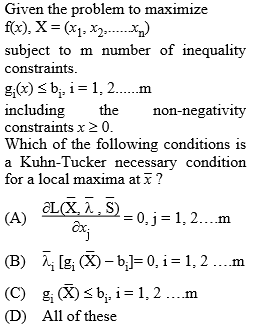Ans: (D)
Q3. The following Linear Programming problem has:
Max              Z = x1 + x2
Subject to
x1 – x2 ≥ 0
3×1 – x2 ≤ –3
and  x1, x2 ≥ 0
(A) Feasible solution
(B) No feasible solution
(C) Unbounded solution
(D) Single point as solution
Ans: (B)
Q4. Given a flow graph with 10 nodes, 13 edges and one connected components, the number of regions and the number of predicate (decision) nodes in the flow graph will be
(A) 4, 5
(B) 5, 4
(C) 3, 1
(D) 13, 8
Ans: (B)
Q5. Function points can be calculated by
(A) UFP ∗ CAF
(B) UFP ∗ FAC
(C) UFP ∗ Cost
(D) UFP ∗ Productivity
Ans: (A)
Q6. Match the following:
 List-I List-II a. Data coupling i. Module A and Module B have shared data b. Stamp coupling ii. Dependency between modules is based on the fact they communicate by only passing of data c. Common coupling iii. When complete data structure is passed from one module to another d. Content coupling iv. When the control is passed from one module to the middle of another
Codes:
 a b c d (A) iii ii i iv (B) ii iii i iv (C) ii iii iv i (D) iii ii iv i
Ans: (B)
Q7. A process which defines a series of tasks that have the following four primary objectives is known as
1. to identify all items that collectively define the software configuration.
2. to manage changes to one or more of these items.
3. to facilitate the construction of different versions of an application.
4. to ensure that software quality is maintained as the configuration evolves over time.
(A) Software Quality Management Process
(B) Software Configuration Management Process
(C) Software Version Management Process
(D) Software Change Management Process
Ans: (B)
Q8. One weakness of boundary value analysis and equivalence partitioning is
(A) they are not effective.
(B) they do not explore combinations of input circumstances.
(C) they explore combinations of input circumstances.
(D) none of the above.
Ans: (B)
Q9. Which once of the following is not a software myth?
(A) Once we write the program and get it to work, our job is done.
(B) Project requirements continually change, but change can be easily accommodated because software is flexible.
(C) If we get behind schedule, we can add more programmers and catch up.
(D) If an organization does not understand how to control software projects internally, it will invariably struggle when it outsources software projects.
Ans: (D)
Q10. Match the following with respect to relationship between objects and classes:
 List-I List-II a. State diagram i. Useful for both abstract modelling and for designing actual program b. Object diagram ii. Describes object classes c. Class diagram iii. Useful for documenting test cases d. Instance diagram iv. Describing the behaviour of a single class of objects.
Codes:
 a b c d (A) iv i ii iii (B) ii iii iv i (C) iii iv ii i (D) ii iv i iii
Ans: (A)
Q11. Match the following style rules for reusability:
 List-I List-II a. Keep methods coherent i. Write a method to get the last element of a list b. Keep methods small ii. Maintain parallel structure when possible c. Keep methods consistent iii. Breaking a method into smaller parts d. Provide uniform coverage iv. Performs a single function or a group of closely related functions.
related functions.
Codes:
a b c d
(A) iv iii ii i
(B) ii i iv iii
(C) iii iv ii i
(D) ii iii iv i
Ans: (A)
Q12. Which is the protocol for performing RPCs between applications in a language and system independent way?
(A) Hyper Text Transmission Protocol (HTTP)
(B) Simple Network Management Protocol (SNMP)
(C) Simple Object Access Protocol (SOAP)
(D) Simple Mail Transfer Protocol (SMTP)
Ans: (C)
Q13. The document that is used by XSLT to indicate, how to transform the elements of the XML document to another format is
(A) HTML page
(B) DOC type procedure
(C) Style sheet
(D) Stored procedure
Ans: (C)
Q14. Which of the following concepts means adding new concepts to a program as it runs?
(A) Data hiding
(C) Dynamic typing
(D) Dynamic binding
Ans: (B)
(A) Virtual polymorphism
(B) Transient polymorphism
(D) Pseudo polymorphism
Ans: (C)
Q16. Match the following with respect to programming languages:
List – I                                                                        List – II
a. Structured Language                                               i. JAVA
b. Non-structured Language                                       ii. BASIC
c. Object Oriented Programming Language            iii. PASCAL
d. Interpreted Programming Language                       iv. FORTRAN
Codes:
a b c d
(A) iii iv i ii
(B) iv iii ii i
(C) ii iv i iii
(D) ii iii iv i
Ans: (A)
Q17. The compiler converts all operands upto the type of the largest operand is called
(A) Type Promotion
(B) Type Evaluation
(C) Type Conversion
(D) Type Declaration
Ans: (A)
Q18. C++ actually supports the following two complete dynamic systems:
(A) One defined by C++ and the other not defined by C.
(B) One defined by C and one specific to C++
(C) Both are specific to C++
(D) Both of them are improvements of C
Ans: (B)
Q19. Important advantage of using new and delete operators in C++ is
(A) Allocation of memory
(B) Frees the memory previously allocated
(C) Initialization of memory easily
(D) Allocation of memory and frees the memory previously allocated.
Ans: (D)
Q20. Match the following control strategies of prolog:
 List-I List-II a. Forward movement i. Variable can be done with a constant, another variable or a function. b. Unification ii. The entire conjunctive goal is executed. c. Deep backtracking iii. Previous sub goal to find alternative solutions. d. Shallow backtracking iv. Chooses sub goal with possible unifier.
Codes:
 a b c d (A) iv i ii iii (B) ii iv i iii (C) iii i iv ii (D) ii iii iv i
Ans: (A)
Q21. Given the following statements:
S1: The grammars S → asb | bsa | ss | a and S → asb | bsa | a are not equivalent.
S2: The grammars S → ss | sss | asb | bsa | λ and S → ss | asb | bsa | λ are equivalent.
Which of the following is true?
(A) S1 is correct and S2 is not correct.
(B) Both S1 and S2 are correct.
(C) S1 is not correct and S2 is correct.
(D) Both S1 and S2 are not correct.
Ans: (A)
Q22. What are the final values of Q1 and Q0 after 4 cock cycles, if initital values are 00 in the sequential circuit shown below: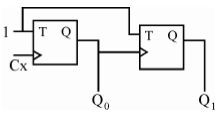(A) 11
(B) 10
(C) 01
(D) 00
Ans: (D)
Q23. High level knowledge which relates to the use of sentences in different contexts and how the context affect the meaning of the sentences?
(A) Morphological
(B) Syntactic
(C) Semantic
(D) Pragmatic
Ans: (D)
Q24. The objective of ________ procedure is to discover at least one ________ that causes two literals to match.
(A) unification, validation
(B) unification, substitution
(C) substitution, unification
(D) minimax, maximum
Ans: (B)
Q25. If  h* represents an estimate of the cost of getting from the current node N to the goal node and h represents actual cost of getting from the current node to the goal node, then A* algorithm gives an optimal solution if
(A) h* is equal to h
(B) h* overestimates h
(C) h* underestimates h
(D) none of these
Ans: (C)
Q26. The mean-end analysis process centers around the detection of differences between the current state and goal state. Once such a difference is isolated, an operator that can reduce the difference must be found. But perhaps that operator cannot be applied to the current state. So a sub-problem of getting to a state in which it can be applied is set up. The kind of backward chaining in which operators are selected and then sub goals are set up to establish the precondition of operators is called
(A) backward planning
(B) goal stack planning
(C) operator subgoaling
Ans: (C)
Q27. In alpha-beta pruning, _________ is used to cut off the search at maximizing level only and _________ is used to cut off the search at minimizing level only.
(A) alpha, beta
(B) beta, alpha
(C) alpha, alpha
(D) beta, beta
Ans: (B)
Q28. If A and B are two fuzzy sets with membership functions
μA(x) = {0.2, 0.5, 0.6, 0.1, 0.9}
μB(x) = {0.1, 0.5, 0.2, 0.7, 0.8}
Then the value of μA ∩ B will be
(A) {0.2, 0.5, 0.6, 0.7, 0.9}
(B) {0.2, 0.5, 0.2, 0.1, 0.8}
(C) {0.1, 0.5, 0.6, 0.1, 0.8}
(D) {0.1, 0.5, 0.2, 0.1, 0.8}
Ans: (D)
Q29. The height h(A) of a fuzzy set A is defined as
h(A) = sup A(x)
x ∈ A
Then the fuzzy set A is called normal when
(A) h(A) = 0
(B) h(A) < 0
(C) h(A) = 1
(D) h(A) < 1
Ans: (C)
Q30. An artificial neuron receives n inputs x1, x2,…., xn with weights w1, w2,…., wn attached to the input links. The weighted sum ________ is computed to be passed on to a non-linear filter φ called activation function to release the output.
(A) Σ wi
(B) Σ xi
(C) Σ wi + Σ xi
(D) Σ wi ⋅ xi
Ans: (D)
Q31. Consider the formula in image processing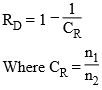CR is called as compression ratio n1 and n2 denotes the number of information carrying units in two datasets that represent the same information. In this situation RD is called as relative _________ of the first data set.
(A) Data Compression
(B) Data Redundancy
(C) Data Relation
(D) Data Representation
Ans: (B)
Q32. Find the false statement:
(A) In Modern Cryptography, symmetric key algorithms use same key both for Encryption and Decryption.
(B) The symmetric cipher DES (Data Encryption Standard) was widely used in the industry for security product.
(C) The AES (Advanced Encryption Standard) cryptosystem allows variable key lengths of size 56 bits and 124 bits.
(D) Public key algorithms use two different keys for Encryption and Decryption.
Ans: (C)
Q33. The message 11001001 is to be transmitted using the CRC polynomial x3 + 1 to protect it from errors. The message that should be transmitted is
(A) 110010011001
(B) 11001001
(C) 110010011001001
(D) 11001001011
Ans: (D)
Q34. _________ comparisons are necessary in the worst case to find both the maximum and minimum of n numbers.
(A) 2n – 2
(B) n + floor (lg n) – 2
(C) floor (3n/2) – 2
(D) 2 lg n – 2
Ans: (C)
Q35. Let A and B be two n × n matrices.
The efficient algorithm to multiply the two matrices has the time complexity
(A) O(n3)
(B) O(n2.81)
(C) O(n2.67)
(D) O(n2)
Ans: (B)
Q36. The recurrence relation T(n)=mT(n/2) tan2 is satisfied by
(A) O(n2)
(B) O(n1g m)
(C) O(n2 lg n)
(D) O(n 1g n)
Ans: (—-)
Q37. The longest common subsequence of the sequences X = and Y = has length
(A) 2
(B) 3
(C) 4
(D) 5
Ans: (C)
Q38. Assuming there are n keys and each key is in the range [0, m – 1]. The run time of bucket sort is
(A) O(n)
(B) O(n lgn)
(C) O(n lgm)
(D) O(n + m)
Ans: (D)
Q39. A _________ complete subgraph and a _________ subset of vertices of a graph G = (V, E) are a clique and a vertex cover respectively.
(A) minimal, maximal
(B) minimal, minimal
(C) maximal, maximal
(D) maximal, minimal
Ans: (D)
Q40. Pumping lemma for context-free languages states:
Let L be an infinite context free language. Then there exists some positive integer m such that any w ∈ L with |w| ≥ m can be decomposed as
w = uv xy Z with |vxy| _________
and |vy| _________ such that uvz xyz
Z ∈ L for all z = 0, 1, 2, ……. .
(A) ≤ m, ≤ 1
(B) ≤ m, ≥ 1
(C) ≥ m, ≤ 1
(D) ≥ m, ≥ 1
Ans: (B)
Q41. The Greibach normal form grammar for the language L = {an bn + 1 | n ≥ 0} is
(A) S → a SB, B → bB | λ
(B) S → a SB, B → bB | b
(C) S → a SB | b, B → b
(D) S → a Sb | b
Ans: (C)
Q42. Given the following statements:
S1 : Every context-sensitive language L is recursive.
S2 : There exists a recursive language that is not context sensitive.
Which statement is correct?
(A) S1 is not correct and S2 is not correct.
(B) S1 is not correct and S2 is correct.
(C) S1 is correct and S2 is not correct.
(D) S1 is correct and S2 is correct.
Ans: (D)
Q43. What is the bit rate for transmitting uncompressed 800 × 600 pixel colour frames with 8 bits/pixel at 40 frames/second?
(A) 2.4 Mbps
(B) 15.36 Mbps
(C) 153.6 Mbps
(D) 1536 Mbps
Ans: (C)
Q44. In IPV 4, the IP address 200.200.200.200 belongs to
(A) Class A
(B) Class B
(C) Class C
(D) Class D
Ans: (C)
Q45. Which layer of OSI reference model is responsible for decomposition of messages and generation of sequence numbers to ensure correct re-composition from end to end of the network?
(A) Physical
(C) Transport
(D) Application
Ans: (C)
Q46. A client-server system uses a satellite network, with the satellite at a height of 40,000 kms. What is the best-case delay in response to a request? (Note that the speed of light in air is 3,00,000 km/second).
(A) 133.33 m sec
(B) 266.67 m sec
(C) 400.00 m sec
(D) 533.33 m sec
Ans: (D)
Q47. The start and stop bits are used in serial communication for
(A) error detection
(B) error correction
(C) synchronization
(D) slowing down the communication
Ans: (C)
Q48. _________ is a type of transmission impairment in which the signal looses strength due to the resistance of the transmission medium.
(A) Attenuation
(B) Distortion
(C) Noise
(D) Decibel
Ans: (A)
Q49. Match the following:
 List-1 List-2 a. Indexed Addressing i. is not used when an operand is moved from memory into a register or from a register to memory. b. Direct Addressing ii. Memory address is computed by adding up two registers plus an (optional) offset. c. Register Addressing iii. Addressing memory by giving a register plus a content offset. d. Base-Indexed Addressing iv. can only be used to access global variables whose address is known at compile time.
Codes:
 a b c d (A) ii i iv iii (B) ii iv i iii (C) iii iv i ii (D) iii i iv ii
Ans: (C)
Q50. Which of the following is a design criteria for instruction formats?
(A) The size of instructions
(B) The number of bits in the address fields
(C) The sufficient space in the instruction format to express all the operations desired.
(D) All of these
Ans: (D)
Q51. Synchronization is achieved by a timing device called a ________ which generates a periodic train of________.
(A) clock generator, clock pulse
(B) master generator, clock pulse
(C) generator, clock
(D) master clock generator, clock pulse
Ans: (D)
Q52. Serial access memories are useful in applications where
(A) Data consists of numbers
(B) Short access time is required
(C) Each stored word is processed differently.
(D) None of these
Ans: (D)
Q53. What will be the output of the following logic diagram?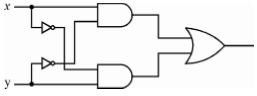(A) xORy
(B) xANDy
(C) xXORy
(D) xXNORy
Ans: (C)
Q54. The essential difference between traps and interrupts is
(A) traps are asynchronous and interrupts are synchronous with the program.
(B) traps are synchronous and interrupts are asynchronous with the program.
(C) traps are synchronous and interrupts are asynchronous with the I/O devices.
(D) None of these.
Ans: (B)

Q55. Consider the ER diagram: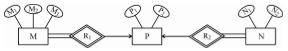The minimum number of tables required to represent M, N, P, R1, R2 is
(A) 2
(B) 3
(C) 4
(D) 5
Ans: (A)
Q56. Consider the following schemas:
Branch = (Branch-name, Assets, Branch-city)
Customer = (Customer-name, Bank name, Customer-city)
Borrow = (Branch-name, loan number, customer account-number)
Deposit = (Branch-name, Account number, Customer-name, Balance)
Using relational Algebra, the Query that finds customers who have balance more than 10,000 is _______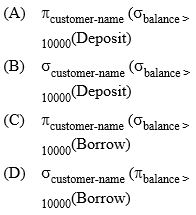Ans: (A)
Q57. Find the false statement:
(A) The relationship construct known as the weak relationship type was defined by Dey, Storey & Barron (1999)
(B) A weak relationship occurs when two relationship types are linked by either Event-Precedent sequence or Condition-Precedent sequence.
(C) Conceptual model is not accurate representation of “Universe of interest”.
(D) Ternary, Quaternary and Quintary relationships are shown through a series of application scenario’s and vignette’s.
Ans: (C)
Q58. Consider the table Student (stuid, name, course, marks).
Which one of the following two queries is correct to find the highest marks student in course 5?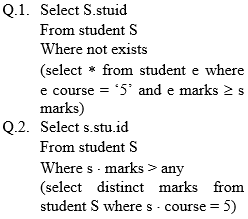(A) Q. 1
(B) Q. 2
(C) Both Q. 1 and Q. 2
(D) Neither Q. 1 nor Q. 2
Ans: (B)
Q59. Armstrong (1974) proposed systematic approach to derive functional dependencies. Match the following w.r.t. functional dependencies:
 List-I List-II a. Decomposition rule i. If X → Y and Z → W then {X, Z} → {Y, W} b. Union rule ii. If X → Y and {Y, W}→Z then {X, W} → Z c. Composition rule iii. If X → Y and X→ Z then X → {Y, Z} d. Pseudo transitivity rule iv. If X → {Y, Z} then X → Y and X → Z
Codes:
 a b c d (A) iii ii iv i (B) i iii iv ii (C) ii i iii iv (D) iv iii I ii
Ans: (D)
Q60. Match the following:
 List-I List-II a. Secondary Index i. Functional Dependency b. Nonprocedural Query Language ii. B-Tree c. Closure of set of Attributes iii. Relational Algebraic Operation d. Natural JOIN iv. Domain Calculus
Codes:
 a b C d (A) i ii Iv iii (B) ii I Iv iii (C) i iii Iv ii (D) ii iv I iii
Ans: (D)
Q61. Which of the following is not true with respect to a trackball and/or spaceball?
I. A trackball is a two dimensional positioning device while as a spaceball provides six degrees of freedom.
II. Unlike the trackball a spaceball does not actually move.
III. A trackball is a three dimensional positioning device while as a spaceball provides six degrees of freedom.
(A) I & II
(B) II & III
(C) II only
(D) III only
Ans: (D)
Q62. Which of the following statement(s) is (are) true?
I. Two successive translations are additive.
II. Two successive rotations are additive.
III. Two successive scaling operations are multiplicative.
(A) I and II
(B) I and III
(C) II and III
(D) All the above
Ans: (D)
Q63. Given below are three basic rules:
I. Squash and Stretch
II. Slow-in and Slow-out
III. To stage the action properly
These rules are applied in case of
(A) Rendering
(B) Morphing
(C) Animation
(D) All the above
Ans: (C)
Q64. Which of the following points lies on the same side as the origin, with reference to the line 3x + 7y = 2?
(A) (3, 0)
(B) (1, 0)
(C) (0.5, 0.5)
(D) (0.5, 0)
Ans: (D)
Q65. The transforming matrix required for conversion of CMY colour model to RGB colour model is given as: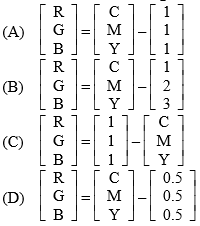Ans: (C)
Q66. What steps shall be required to rotate an object about the point P1 (as shown in Fig. 1) and its placement such that what was at P2 (as shown in Fig. 2)?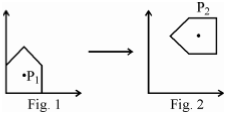I. Translate P1 to origin
II. Scale as required
III. Rotate
IV. Translate to the final position P2.
(A)  I, II and III
(B) II, III and IV
(C) I, III and IV
(D) All of the above
Ans: (D)
Q67. In Unix, how do you check that two given strings a and b are equal?
(A) test \$a –eq \$b
(B) test \$a –equal \$b
(C) test \$a = \$b
(D) Sh –C test \$a = = \$b
Ans: (C)
Q68. In windows 2000 operating system all the processor-dependent code is isolated in a dynamic link library called
(A) NTFS file system
(B) Hardware abstraction layer
(C) Microkernel
(D) Process Manager
Ans: (B)
Q69. To place a sound into a word document, following feature of windows is used:
(A) Clip board
(C) C Win App
(D) OLE
Ans: (D)
Q70. Translation Look-aside Buffer (TLB) is
(A) a cache-memory in which item to be searched is compared one-by-one with the keys.
(B) a cache-memory in which item to be searched is compared with all the keys simultaneously.
(C) an associative memory in which item to be searched is compared one-by-one with the keys.
(D) an associative memory in which item to be searched is compared with all the keys simultaneously.
Ans: (D)
Q71. Simplest way of deadlock recovery is
(A) Roll back
(B) Preempt resource
(C) Lock one of the processes
(D) Kill one of the processes
Ans: (D)
Q72. The directory structure used in Unix file system is called
(A) Hierarchical directory
(B) Tree structured directory
(C) Directed acyclic graph
(D) Graph structured directory
Ans: (C)
Q73. Which statement is not true about process O in the Unix operating system?
(A) Process O is called init process.
(B) Process O is not created by fork system call.
(C) After forking process 1, process O becomes swapper process.
(D) Process O is a special process created when system boots.
Ans: (A)
Q74. Which of the following commands would return process_id of sleep command?
(A) Sleep 1 and echo \$?
(B) Sleep 1 and echo \$#
(C) Sleep 1 and echo \$×
(D) Sleep 1 and echo \$!
Ans: (D)
Q75. Possible thread states in Windows 2000 operating system include: